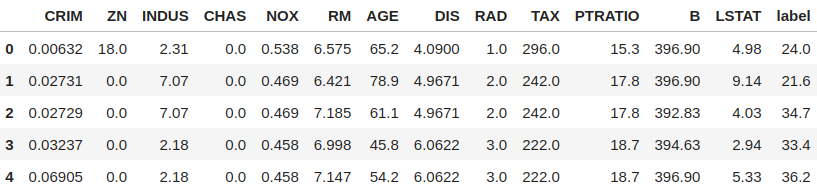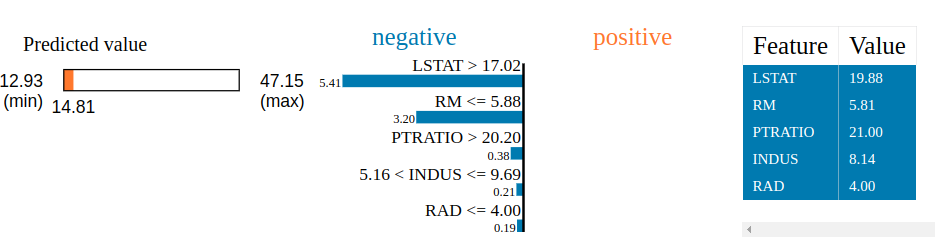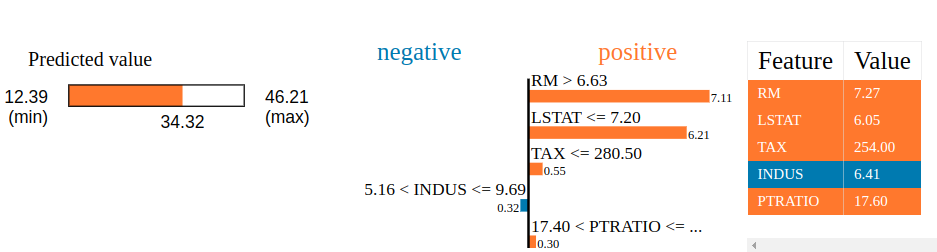GeeksforGeeks App
Open AppBrowser
Continue

## Related Articles

The vast field of Artificial Intelligence(AI) has experienced enormous growth in recent years. With newer and more complex models coming each year, AI models have started to surpass human intellect at a pace that no one could have predicted. But as we get more accurate and precise results, it’s becoming harder to explain the reasoning behind the complex mathematical decisions these models take. This mathematical abstraction also doesn’t help the users maintain their trust in a particular model’s decisions.

e.g., Say a Deep Learning model takes in an image and predicts with 70% accuracy that a patient has lung cancer. Though the model might have given the correct diagnosis, a doctor can’t really advise a patient confidently as he/she doesn’t know the reasoning behind the said model’s diagnosis.

Here’s where Explainable AI(XAI) comes in.

## Explainable AI(XAI)

Explainable AI collectively refers to techniques or methods, which help explain a given AI  model’s decision-making process. This newly found branch of AI has shown enormous potential, with newer and more sophisticated techniques coming each year. Some of the most famous XAI techniques include SHAP (Shapley Additive exPlanations), DeepSHAP, DeepLIFT, CXplain, and LIME. This article covers LIME in detail.

## LIME (or Local  Interpretable Model-agnostic Explanations)

The beauty of LIME its accessibility and simplicity. The core idea behind LIME though exhaustive is really intuitive and simple! Let’s dive in and see what the name itself represents:

• Model agnosticism refers to the property of LIME using which it can give explanations for any given supervised learning model by treating it as a ‘black box’ separately. This means that LIME can handle almost any model that exists out there in the wild!
• Local explanations mean that LIME gives explanations that are locally faithful within the surroundings or vicinity of the observation/sample being explained.

Though LIME limits itself to supervised Machine Learning and Deep Learning models in its current state, it is one of the most popular and used XAI methods out there. With a rich open-source API, available in R and Python, LIME boasts a huge user base, with almost 8k stars and 2k forks on its GitHub repository [https://github.com/marcotcr/lime]

### How LIME works?

Broadly speaking, when given a prediction model and a test sample, LIME does the following steps:

• Sampling and obtaining a surrogate dataset: LIME provides locally faithful explanations around the vicinity of the instance being explained. By default, it produces 5000 samples(see the num_samples variable) of the feature vector following the normal distribution. Then it obtains the target variable for these 5000 samples using the prediction model, whose decisions it’s trying to explain.
• Feature Selection from the surrogate dataset: After obtaining the surrogate dataset, it weighs each row according to how close they are to the original sample/observation. Then it uses a feature selection technique like Lasso to obtain the top important features.

LIME also employs a Ridge Regression model on the samples using only the obtained features. The output prediction should theoretically be similar in magnitude to the one output by the original prediction model. This is done to stress the relevance and importance of these obtained features.

#### Installing LIME

Coming to the installation part, we can use either pip or conda to install LIME in Python.

pip install lime

or

conda install -c conda-forge lime

Before going ahead, here are some key pointers that would help gain a much better understanding of the whole workflow surrounding LIME.

### Dataset Description:

LIME in its current state is only able to give explanations for the following type of datasets:

1. Tabular datasets (lime.lime_tabular.LimeTabularExplainer): eg: Regression, classification datasets
2. Image-related datasets (lime.lime_image.LimeImageExplainer)
3. Text-related datasets (lime.lime_text.LimeTextExplainer)

Since this is an introductory article, we’ll keep things simple and go ahead with a tabular dataset. More specifically, we’ll be using the Boston House Pricing dataset for our analysis. We’ll be using the Scikit-Learn utility for loading the Boston dataset.

### Prediction Model Used:

As LIME is model agnostic in nature, it can handle almost any model thrown at it. To stress this fact, we’ll be using an Extra-trees regressor through the Scitkit-learn utility as our prediction model whose decisions we’re trying to investigate.

### Brief Introduction to LimeTabularExplainer

As explained above, we’ll be using a tabular dataset for our analysis. To tackle such datasets, LIME’s API offers the LimeTabularExplainer.

Syntax: lime.lime_tabular.LimeTabularExplainer(training_data, mode,  feature_names, verbose)

Parameters:

• training_data – 2d array consisting of the training dataset
• mode – Depends on the problem; “classification” or “regression”
• feature_names – list of titles corresponding to the columns in the training dataset. If not mentioned, it uses the column indices.
• verbose – if true, print local prediction values from the regression model trained on the samples using only the obtained features

Once instantiated, we’ll use a method from the defined explainer object to explain a given test sample.

Syntax: explain_instance(data_row, predict_fn, num_features=10, num_samples=5000)

Parameters:

• data_row – 1d array containing values corresponding to the test sample being explained
• predict_fn – Prediction function used by the prediction model
• num_features – maximum number of features present in explanation
• num_samples – size of the neighborhood to learn the linear model

For the sake of brevity and conciseness, only some of the arguments have been mentioned in the above two syntaxes. The rest of the arguments, most of which default to some cleverly optimized values, can be checked out by the interested reader at the official LIME documentation[https://lime-ml.readthedocs.io/en/latest/]

Workflow

1. Data preprocessing
2. Training an Extra-trees regressor on the dataset
3. Obtaining explanations for a given test sample

Analysis

## Python

 # Importing the necessary librariesimport numpy as npimport matplotlib.pyplot as pltimport pandas as pd # Loading the dataset using sklearnfrom sklearn.datasets import load_bostondata = load_boston() # Displaying relevant information about the dataprint(data['DESCR'][200:1420])

Output:

Boston house prices dataset
---------------------------

**Data Set Characteristics:**

:Number of Instances: 506

:Number of Attributes: 13 numeric/categorical predictive. Median Value (attribute 14) is usually the target.

:Attribute Information (in order):
- CRIM     per capita crime rate by town
- ZN       proportion of residential land zoned for lots over 25,000 sq.ft.
- INDUS    proportion of non-retail business acres per town
- CHAS     Charles River dummy variable (= 1 if tract bounds river; 0 otherwise)
- NOX      nitric oxides concentration (parts per 10 million)
- RM       average number of rooms per dwelling
- AGE      proportion of owner-occupied units built prior to 1940
- DIS      weighted distances to five Boston employment centres
- TAX      full-value property-tax rate per $10,000 - PTRATIO pupil-teacher ratio by town - B 1000(Bk - 0.63)^2 where Bk is the proportion of black people by town - LSTAT % lower status of the population - MEDV Median value of owner-occupied homes in$1000's

:Missing Attribute Values: None

:Creator: Harrison, D. and Rubinfeld, D.L.

This is a copy of UCI ML housing dataset.
https://archive.ics.uci.edu/ml/machine-learning-databases/housing/

## Python

 # Separating data into feature variable X and target variable y respectivelyfrom sklearn.model_selection import train_test_splitX = data['data']y = data['target'] # Extracting the names of the features from datafeatures = data['feature_names'] # Splitting X & y into training and testing setX_train, X_test, y_train, y_test = train_test_split(    X, y, train_size=0.90, random_state=50) # Creating a dataframe of the data, for a visual checkdf = pd.concat([pd.DataFrame(X), pd.DataFrame(y)], axis=1)df.columns = np.concatenate((features, np.array(['label'])))print("Shape of data =", df.shape) # Printing the top 5 rows of the dataframedf.head()

Output:

Shape of data = (506, 14)## Python

 # Instantiating the prediction model - an extra-trees regressorfrom sklearn.ensemble import ExtraTreesRegressorreg = ExtraTreesRegressor(random_state=50) # Fitting the predictino model onto the training setreg.fit(X_train, y_train) # Checking the model's performance on the test setprint('R2 score for the model on test set =', reg.score(X_test, y_test))

Output:

R2 score for the model on test set = 0.9183847734063736

## Python

 # Importing the module for LimeTabularExplainerfrom lime import lime_tabular # Instantiating the explainer object by passing in the training set,# and the extracted featuresexplainer_lime = lime_tabular.LimeTabularExplainer(X_train,                                                   feature_names=features,                                                   verbose=True,                                                   mode='regression')

#### 5. Getting explanations by calling the explain_instance() method

• Suppose we want to explore the prediction model’s reasoning behind the prediction it gave for the i’th test vector.
• Moreover, say we want to visualize the top k features which led to this reasoning.

For this article, we’ve given explanations for two combinations of i & k:

#### 5.1 Explaining the decisions for i=10, k=5

We’re basically asking LIME to explain the decisions behind the predictions for the 10th test vector by displaying the top 5 features which contributed towards the said model’s prediction.

## Python

 # Index corresponding to the test vectori = 10 # Number denoting the top featuresk = 5 # Calling the explain_instance method by passing in the:#    1) ith test vector#    2) prediction function used by our prediction model('reg' in this case)#    3) the top features which we want to see, denoted by k exp_lime = explainer_lime.explain_instance(    X_test[i], reg.predict, num_features=k) # Finally visualizing the explanationsexp_lime.show_in_notebook()

Output:

Intercept 20.03385472541795
Prediction_local [33.9748055]
Right: 34.323999999999984LIME XAI  explanations

Interpreting the output:

There’s plenty of information that LIME outputs! Let’s go step by step and interpret what it’s trying to convey

1. First off, we see three values just above the visualizations:

1. Right: This denotes the prediction given by our prediction model (an extra-trees regressor in this case) for the given test vector.
2. Prediction_local: This denotes the value outputted by a linear model trained on the perturbed samples (obtained by sampling around the test vector following a normal distribution) and using only the top k features outputt by LIME.
3. Intercept: The intercept is the constant part of the prediction given by the above linear model’s prediction for the given test vector.2. Coming to the visualizations, we can see the colors blue and orange, depicting negative and positive associations, respectively.

• To interpret the above results, we can conclude that the relatively lower price value(depicted by a bar on the left) of the house depicted by the given vector can be attributed to the following socio-economic reasons:
• The high value of LSTAT indicates the lower status of a society in terms of education and the population
• The high value of PTRATIO indicates the high value of the number of students per teacher
• The high value of INDUS indicates the high proportion of non-retail business acres per town
• The low value of RAD indicates a less index of accessibility to radial highways
• The low value of RM indicates the less amount of room per dwelling

We can see how easy it has become to correlate the decisions taken by a relatively complex prediction model(an extra-trees regressor) in an interpretable and meaningful way. Let’s try this exercise on one more test vector!

#### 5.2 Explaining the decisions for i=47, k=5

Here again, we’re asking LIME to explain the decisions behind the predictions for the 47th test vector by displaying the top 5 features which contributed towards the said model’s prediction

## Python

 # Index corresponding to the test vectori = 47 # Number denoting the top featuresk = 5 # Calling the explain_instance method by passing in the:#    1) ith test vector#    2) prediction function used by our prediction model('reg' in this case)#    3) the top features which we want to see, denoted by kexp_lime = explainer_lime.explain_instance(    X_test[i], reg.predict, num_features=k) # Finally visualizing the explanationsexp_lime.show_in_notebook()

Output:

Intercept 20.03666971464815
Prediction_local [33.88485397]
Right: 34.323999999999984XAI LIME  explanations

Interpreting the output:

From the visualizations, we can conclude that the relatively higher price value (depicted by a bar on the left) of the house depicted by the given vector can be attributed to the following socio-economic reasons:

• The low value of LSTAT  indicates the grand status of a society in terms of population, education, and employability
• The high value of RM indicates the high number of rooms per dwelling
• The low value of TAX indicates the low tax rate of the property
• The low value of PTRATIO indicates a good number of students per teacher
• The low value of AGE which depicts the newness of the establishment
• The average value of INDUS indicates that the low number of non-retails near the society, has decreased the value of the house to a small extent.

### Summary:

This article is a brief introduction to Explainable AI(XAI) using LIME in Python. It’s evident how beneficial LIME could give us a much more profound intuition behind a given black-box model’s decision-making process while providing solid insights on the inherent dataset. This makes LIME a useful resource for both AI researchers and data scientists alike!

My Personal Notes arrow_drop_up